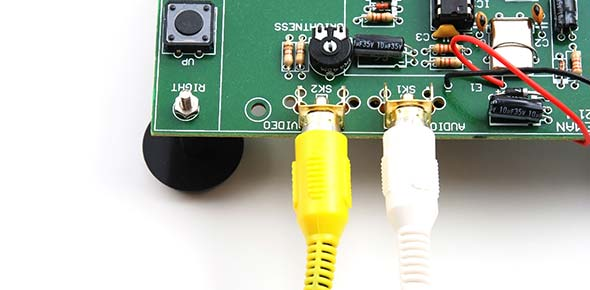# Electronics Engineering Toughest Quiz!

20 Questions | Total Attempts: 31046Settings.

Related Topics
• 1.
Of the following substances, the best conductor is
• A.

Air

• B.

Copper

• C.

Iron

• D.

Salt water

• 2.
The atomic number of an element is determined by?
• A.

the number of neutrons

• B.

the number of protons

• C.

the number of neutrons plus the number of protons

• D.

the number of electrons

• 3.
The atomic weight of an element is approximately determined by?
• A.

the number of neutrons

• B.

the number of protons

• C.

the number of neutrons plus the number of protons

• D.

the number of electrons

• 4.
Suppose there is an atom of oxygen, containing eight protons and eight neutrons in the nucleus, and two neutrons are added to the nucleus. What is the resulting atomic weight?
• A.

18

• B.

8

• C.

10

• D.

16

• 5.
An ion
• A.

Is electrically neutral

• B.

Has positive electric charge

• C.

Has negative electric charge

• D.

Can have either a positive or negative charge

• 6.
An isotope
• A.

Is electrically neutral

• B.

Has positive electric charge

• C.

Has negative electric charge

• D.

Can have either a positive or negative charge

• 7.
A molecule
• A.

Can consist of a single atom of an element

• B.

Always contains two or more elements

• C.

Always has two or more atoms

• D.

Is always electrically charged

• 8.
In a compound,
• A.

There can be a single atom of an element

• B.

There must always be two or more elements

• C.

The atoms are mixed in with each other but not joined

• D.

There is always a shortage of electrons

• 9.
An electrical insulator can be made a conductor
• A.

By heating it

• B.

By cooling it

• C.

By ionizing it

• D.

By oxidizing it

• 10.
Of the following substances, the worst conductor is
• A.

Air

• B.

Copper

• C.

Iron

• D.

Salt water

• 11.
Movement of holes in a semiconductor
• A.

Is like a flow of electrons in the same direction

• B.

Is possible only if the current is high enough

• C.

Results in a certain amount of electric current

• D.

Causes the material to stop conducting

• 12.
If a material has low resistance, then
• A.

It is a good conductor

• B.

It is a poor conductor

• C.

The current flows mainly in the form of holes

• D.

Current can flow only in one direction

• 13.
A coulomb
• A.

Represents a current of 1 ampere

• B.

Flows through a 100-watt light bulb

• C.

Is equivalent to 1 ampere per second

• D.

Is an extremely large number of charge carriers

• 14.
A stroke of lightning
• A.

Is caused by a movement of holes in an insulator

• B.

Has a very low current

• C.

Is a discharge of static electricity

• D.

Builds up between clouds

• 15.
The volt is the standard unit of
• A.

Current

• B.

Charge

• C.

Electromotive force

• D.

Resistance

• 16.
If an EMF of 1 volt is placed across a resistance of 2 ohms, then the current is
• A.

Half an ampere

• B.

1 ampere

• C.

2 amperes

• D.

Impossible to determine

• 17.
A backward-working electric motor, in which mechanical rotation is converted to electricity,
• A.

An inefficient, energy-wasting device

• B.

A motor with the voltage connected the wrong way

• C.

An electric generator

• D.

A magnetic field

• 18.
In a battery, chemical energy can sometimes be replenished by
• A.

Connecting it to a light bulb

• B.

Charging it

• C.

Discharging it

• D.

No means known; when a battery is dead, you must throw it away

• 19.
A fluctuating magnetic field
• A.

Produces an electric current in an insulator

• B.

Magnetizes the earth

• C.

Produces a fluctuating electric field

• D.

Results from a steady electric current

• 20.
Visible light is converted into electricity
• A.

in a dry cell

• B.

In a wet cell

• C.

In an incandescent bulb

• D.

In a photovoltaic cell